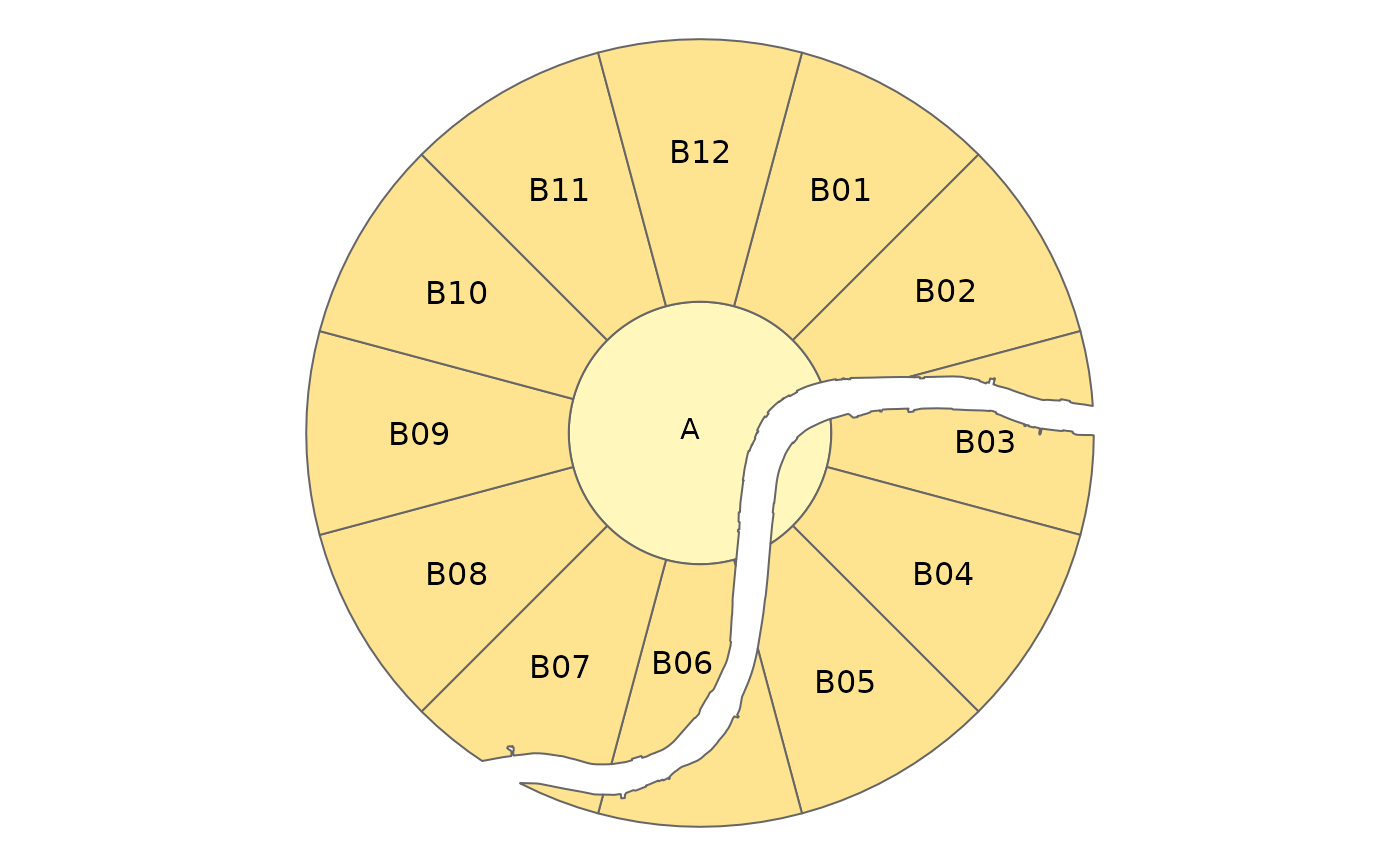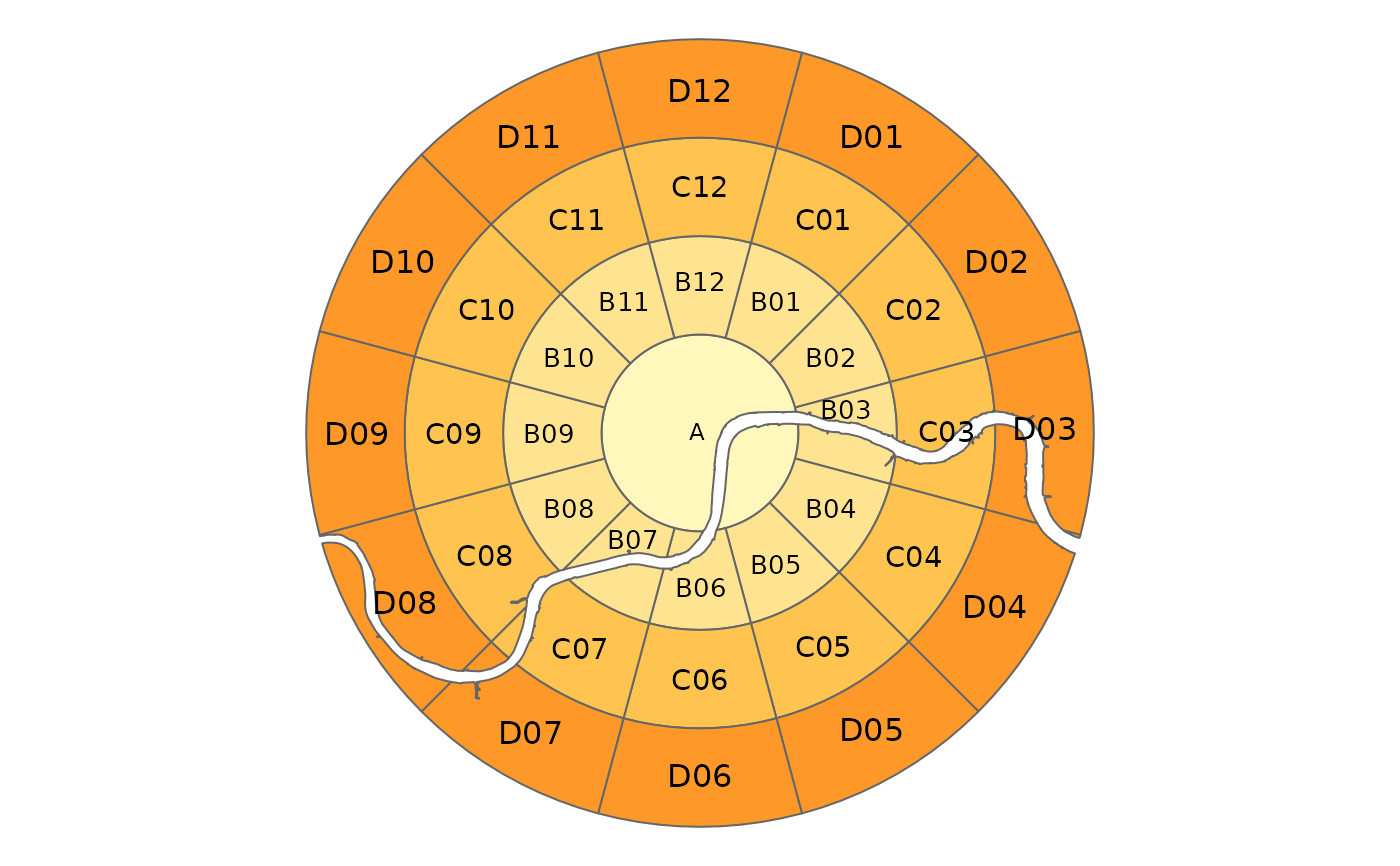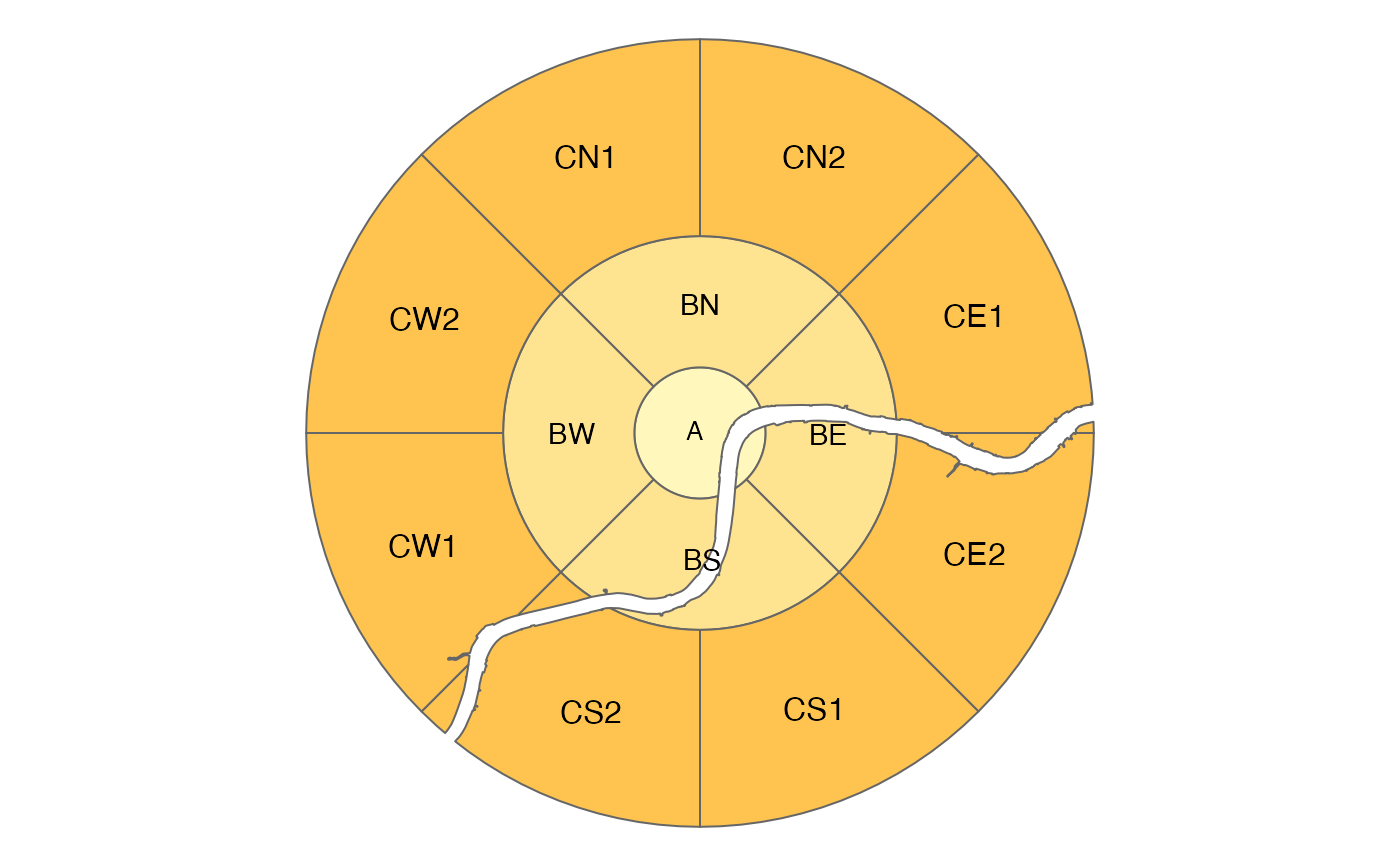This function first divides geographic space into [annuli](https://en.wikipedia.org/wiki/Annulus_(mathematics)) (concentric 2d rings or 'doughnuts') and then subdivides each annulus into a number of segments.

zb_zone(
x = NULL,
area = NULL,
n_circles = NA,
n_segments = 12,
distance = 1,
distance_growth = 1,
labeling = NA,
starting_angle = NA,
segment_center = FALSE,
intersection = TRUE,
city = NULL
)

Arguments

x

Centre point. Should be an sf or sfc object containing one point, or a name of a city (which is looked up with OSM geocoding).

area

(optional) Area. Should be an sf or sfc object containing one (multi) polygon

n_circles

Number of rings including the central circle. By default 5, unless area is specified (then it is set automatically to fill the area).

n_segments

(optional) Number of segments. The number of segments. Either one number which determines the number of segments applied to all circles, or a vector with a number for each circle (which should be a multiple of 4, see also the argument labeling). By default, the central circle is not segmented (see the argument segment_center).

distance

Distance The distances between the circles. For the center circle, it is the distance between the center and the circle. If only one number is specified, distance_growth determines the increment at which the distances grow for the outer circles.

distance_growth

The rate at which the distances between the circles grow. Only applicable when distance is one number and n_circles > 1. See also distance.

labeling

The labeling of the zones. Either "clock" which uses the clock ananolgy (i.e. hours 1 to 12) or "NESW" which uses the cardinal directions N, E, S, W. If the number of segments is 12, the clock labeling is used, and otherwise NESW. Note that the number of segments should be a multiple of four. If, for instance the number of segments is 8, than the segments are labeled N1, N2, E1, E2, S1, S2, W1, and W2.

starting_angle

The angle of the first of the radii that create the segments (degrees). By default, it is either 15 when n_segments is 12 (i.e. the ClockBoard setting) and -45 otherwise.

segment_center

Should the central circle be divided into segments? FALSE by default.

intersection

Should the zones be intersected with the area? TRUE by default.

city

(optional) Name of the city. If specified, it adds a column city to the returned sf object.

Value

An sf object containing zones covering the region

Details

By default 12 segments are used for each annuli, resulting in a zoning system that can be used to refer to segments in [clock position](https://en.wikipedia.org/wiki/Clock_position), with 12 representing North, 3 representing East, 6 Sounth and 9 Western segments.

Examples

# default settings
z = zb_zone(london_c(), london_a())
# \donttest{
zb_plot(z)if (require(tmap)) {
zb_view(z)

z = zb_zone("Berlin")
zb_view(z)
}